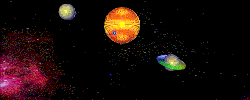P L A N E T A R Y   M O T I O N

Chapter 14INDEX TO OTHER PAGES

The waters rise

1.   The gravitational pull of the earth is more than twice as great as that of our moon, how therefore shall a tug of war be won by the weaker to lift the waters of our ocean, and that at a distance?

2. The answer is of course no, it cannot be the moon's gravity.  Mathematics alone confirms that hovering the moon as near as inches from our ocean surface would in no way cause our tides.  Yet how then did we come to say that the moon's gravity raises our waters?

3. We seem to be good at circumventing problems, since then is was taught that; "The gravitational attraction is itself only small crediting the rest to - traction forces.”

4. These of course are lies, since gravitational attraction is --32.14 at sea level, and there are no traction forces.  And knowing gravity to be pulling -- downwards  -- how do the waters rise -- upwards -- by the same?  Seems to me someone's alcohol level was way up not able to walk a straight line, or count backwards from ten.

5.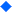But let us survey this, let us set some numbers to say that the gravity of the moon is equal to 3 compared to that of the earth at 10.  The gravitational pull of the moon upon us therefore is not only small, but in fact is 7 points short of coming equal.  We therefore are quite incorrect to say that there is a small gravitational pull of the moon on our waters.

6. Moreover, the gravity of the earth does not even extend ten percent outwards in its distance from the moon.  And that of the moon is even less.  How then speak of gravity in conjunction with the tides?

7. And as to those traction forces, our first question will be: "What traction forces?"  Are we not claiming that planets are bound gravitational?  How therefore shall a thousand lb. traction force pull along a sled into the millions of lb. with still another traction force pulling in reverse at better than twice that amount?

8. Moreover, the ideal of a traction force also requires a - dam - against which the waters may be resisted and - who - shall be holding that dam?

9. If we state that the waters are caused by the very rotation of the earth, the waters being slow to react to that rotation, how, may I ask, will we explain the tides to occur under the moon, and, away from the moon?  For in that case the moon has no relevance at all to our tides.

10. With the earth moving eastwards it is logical that the waters should continually built up on the west-banks.  Our waters however do in no way lag behind in the movement of our daily rotation since our rotation is constant

11. There is no acceleration in our daily rotation, and only by an acceleration or a deceleration can the waters gain upon any one side or the other.

12.Newton came to say that gravity holds all stars and planets.  And somehow, he or we had to explain the tides to our children.  Since gravity then was the only source of any pull we knew of, we had to give it some definition.  I know we have not been happy with it, for even in our textbooks it states that the tides occur contrary to common sense.

13. This now, as if this was not yet bad enough to teach our children, I read a new one lately where we must conceive the earth to be like a ball floating in a sea of water.

14. Arrow A of figure 14-1 displays the orbital track of the earth.  According to this latest theory then, the earth is to physically move out from under its waters so that it may lag behind our movement, which (as they erroneously speculate) causes the tides.

15. That which is very wrong here is for one; that the same would cause only be a single tide on that illogic proposition.  Secondly, having lived on this earth for a number of years I have yet to see the days grow shorter.

16. For what I mean to say with that is - do we not realize how it requires an "acceleration" of the whole earth for such a thing to be even remotely possible?  Or if we (conveniently) presume that the tides are only at right angle our orbital movement - so as not to impair our yearly timetable - may I then ask how they propose to "accelerate", and "decelerate" the whole earth on a daily basis?

17.Let us be reasonable, if we do not quickly swing to the right and left like a pendulum, there would be no acceleration to account for.

18. And how may I ask; will we keep enough stimulant against motion sickness on hand to supply the whole world of men and beast?  I was sick for 8 of the 10 days on my first boat-ride, I don't think I could take that for much longer.

19.Not one of the men whom proposed such theories will of course come with any mathematics to substantiate their claim. And why then should we be fooled by them?

20. As I noted when I pour out a cup of water, it wastes no time in getting to the ground.  It is therefore safe to conclude that the earth has no intentions of leaving its water a track or two behind.Back to Newton.

1.   Coming back to Newton and his theory, there is still this little snag - for the waters to rise on both sides of the earth equally and simultaneously - while the moon is only on one side thereof.  This then is explained in this manner:

2. Reference figure 14-2:  Quote: "The moon pulls more on the waters at point E, supplementing the centrifugal force which tends to cast the water outwards.  And pulling less on the waters at point H where the centrifugal pull exceeds the moon's gravitational pull."

3.   I now am bound to ask: What shall be the difference between "supplementing" and "exceeding" gravitational pull - since both add?  And, what is it to say, - Centrifugal exceeds the moon's gravity?  For does not everything upon us exceeds the minute gravity of the moon?

4. The pull of the moon may be a value of three (3) at its surface, but long before it comes halfway to us - it has left zero for some degree in reverse keeping the waters to the earth down  - instead of lifting them.

5.But rather than using logic alone, let us experiment with values. (Illustration figure 14-2).   Let the centrifugal impact be 20, with the moon's gravitational pull at point E, 30, while at H it shall be 10.  (For the moment we are thus giving Newton the benefit of the doubt)

6. The supplementing force then adds 20 to 30 giving the waters a rise equal to 50.  Then, on the far side (H) the centrifugal 20 exceeds the moon's pull of 10 leaving a net waters rise of only 10, or 1/5th compared to the other side.

7.Obviously, this theory of Newton contradicts itself rather than confirming the facts as we experience them.  And if we care to dig ourselves yet deeper in this quicksand, we can quote our scientist to say., "That the bottom of the ocean near the moon is pulled more than the bottom at the far side".

8. If thus we incorporate these values, giving the ocean bottom at E a gift of 10, causing the rise to be 60.  (Bottom pulled upwards, water rising).  And on the far side (H) we give it a half value, which will be towards the moon also, pulling the ocean bottom in, lowering the tide, which would finalize the tide at that far side to a height of only 5 as compared to 60 under the moon.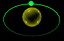Gravity verses centrifugal

1.   Before I enter upon how the tides do indeed occur allow me to lead up to it by defining "gravity verses centrifugal action."  Taking inventory; there is but one fundamental force in nature, which is "magnetism" as in "magnetic force."

2. A division of this force then leads to electrical current as a derivative of this force of magnetic, and gravity as a sister to this masculine force of magnetic.  Next to these there is what we call - a centrifugal impact - which is often referred to as a force.

3. Illustration figure 14-3:   Let X be the center of the earth, and Y a particle of mass held by G (for gravity), and C (for centrifugal).  If for example, the net value of G is 7, and C is 3, the pull of G on mass Y - is not 7, but 10.  For the value of 7 cannot be found except by subtracting C from G. Gravity therefore must have a - gross - value of 10 in order that the net - value be 7.

4.   A simple mathematical solution yes, but do we in fact realize the implications of this simple mathematical solution?  We mind you have just placed a great many men in the wrong with one simple mathematical computation the evidence of which is unassailable.  And so let us consider how this thing can have such devastating implications upon man's conceptions.

5.In our textbooks we state that the centrifugal force is greatest at our equator.  And this being so, it means that as we come nearer to a pole the force of centrifugal decreases.  Yet in all this, a factor called "weight" remains the same.

6. Consequently the power of gravitational pull must vary proportionally with the centrifugal impact.  This therefore strongly suggests the latter to have a direct bearing on the power of gravity.  Weight therefore concludes as gravitational force to always be greater to the centrifugal force.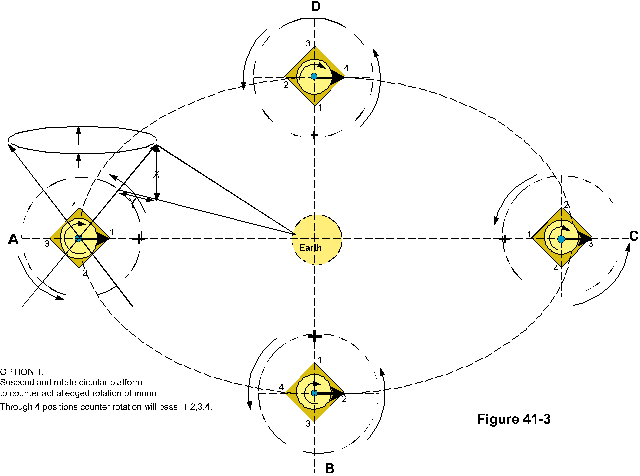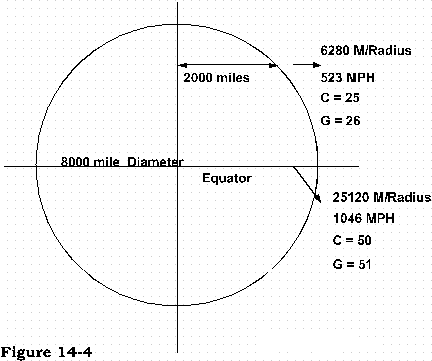1.  I now have said what I needed to say, and we could go on to the next phase.  But since this thing has been left unrealized for so long by so many, I judge it justified to linger a while.

2. For a first, are we sure that 3 plus 7 equals to 10?  If indeed we are, let this therefore be a fact.  How then did we obtain the 7?  We said to let the "net" value of G be 7, and since "net" means, that which is left over from a gross total, the "net" is what we come to weigh on the scales.

3. If therefore the scale reads our weight at 175 lb. it means that we are being pulled to the earth with a force of that net outcome.  It does not mean that gravity is upon us at 175 lb. since we must add the centrifugal impact, which is in direct opposite thereto.  If the centrifugal force causes us to flee from center by 100 lb. the gravitational force must be 275 lb.

4.   Next, at leaving our equator for some point near a polar cap, if the centrifugal impact is reduced to 40 lb. - the gravitational attraction will likewise decrease by that value, and again we will weight the same 175 lb. on the scale.

5. And now stop for a minute, take a good look. Notice how when the centrifugal increases so does gravity!!  What therefore is the logical implication?

6. Is not centrifugal a factor of movement? As therefore movement increases so does the pull of gravity. Might it therefore be that  MOVEMENT IS THE VERY CAUSE TO GRAVITY?

7. Now in order to be absolutely sure of what we are doing, let us compare the laws of gravity and of centrifugal.  The textbook states:

8. “The centrifugal force is directly proportional to the spherical velocity squared, and inversely proportional to the radius of its path.   (True)

And for gravity:

1. "The centripetal (gravity) acceleration of a particle in uniform circular motion is proportional to the square of its speed, and inversely proportional to the radius of its path."  (the term acceleration here is wrong)

2.Spherical velocity now, is the same as, speed, and both laws require that speed to be squared.  The two laws therefore are identical, except in the direction in which they apply.

3. But how is it possible for us to write these things in our textbooks, and, at the same time also state Newton's law of gravity that every particle attracts every other particle according to their volume mass?  For quite obviously these laws are not compatible, they are in fact in direct contradiction with each other.

4.   And so I begin to wonder who it might be that we adhere to. Is it Newton's view, or do we rather adhere to the laws which were established by factual data and experiment?

5. My estimate of all this is, that man has yet to realize the words he has spoken, he has yet to correlate more than one factor at the time, he does not understand his own speech.  It is therefore that I linger.

6.   And so to make the law simple.  It in effect states that, doubling mass doubles force.  Our present concern is not however in how much mass - as it is in the force - which is pronounced upon it.  We will thus deal with just 1 lb., of force in our example.

7.If we cast something around us at a given velocity, it effects a force, and to double that velocity will result into four times (squared) its force.  (When velocity is x 2, force is x 4).

8. An increase in radius however slows down the mass in respect to its vector (center) point, wherefore a doubling of the radius must reduce the force by the half by which it was slowed.  Consequently 2 x radius, equals force divided by 2.

9.   For simplicity let us view just two points on the face of the earth.    By illustration figure 14-4, the 8,000 mile diameter of the earth at its equator computes to some 25,120 miles of circumference, which - in one turn for 24 hours - comes to a velocity of 1,046 miles per hour.

10. At a half point, we at that point - would be traveling at only 523 miles per hour, which is at a radius of 4,000 miles or 2,000 from the axis of the earth's rotation.

11.For the example then let us give the centrifugal impact at the half way point a value of 25 lb. the velocity being 523 mph.  At our equator, the velocity being times 2, the force must be squared (4 times 25) is 100 lb.

12. This in turn must be divided for the double radius (: 2) leaves 50 lb., of centrifugal force.  Our 1 lb., weight - at the equator - has thus a gravitational attraction of 51 lb., while the same 1 lb., at the halfway point - has only a 26 lb., gravitational attraction.

13.   There is no other explanation nor determination that can be made here according to the laws of nature which we ourselves have certified.  We might thus convene a congressional hearing to railroad our scales into scapegoats, since by them we were inclined to sight deception.

14. But then again, are we not more than our scales?  Nor would it be fair to place Newton on the chair, since we ourselves have long been contradicting him.

15. Now however I must admit this to you, that I myself did not learn all this about gravity by the logic as I here defined to you, but rather being as ignorant as everyone else, I sat down one evening and started to write down the cause and nature to gravity and the foundations of the earth without knowing what I was writing.

16. It was like my hand was writing that which it was compelled to write - but the same was not of my mind, nor out of my knowledge, or logic as I displayed here.   For here the Lord clearly wished it shown and understood that these things came to me in a revelation by His Holy Spirit.

17. This was so since it is written by Jeremiah how if any man can discover the foundations of the earth, He the Lord would not save any person from their sins.  Therefore did the Lord give it to me in just that manner to clearly show how it was revealed to me of Him.

18. While in many other things He the Lord, like He did unto Solomon, gave me a spirit of wisdom and of understanding by which I came to define so many things, beside the many secrets which He revealed to me in what men call religious matters.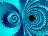Magnetic potential and design

1.   Either a circular or a triangular fashion (Figure 14-5 and 14-6) can draw a magnetic potential.  Both display the general line of motion, which is also the centerline and axis of rotation.

2. The lines crossing each other define the direction of motion in their figure eight, thus called "defined lines of motion".  The intersection of the equatorial line locates the center of force, while the angular lines are merely called "lines of motion".

1. The value of this 3M (3M = Magnetic motion) force then is relative to, (a), the quantity of the mass (inertia)., and (b), the nature of the mass (magnetic value)., and  (c), its movement (rotation etc.)., and (d), The potential of its driver (the sun).

2. Contrary to anything that we may have heard, it is by magnetic force, by magnetic lines, and by magnetic potential that all planets are held to their stars.

3. By these do the planets interact with one another and no other force exists, nor is there any other force capable of holding on to objects of such dimensions.

4. If this is not so evident, then show me just one other force in all of nature, and I will again show you how "it" is magnetic.  Point out gravity, and I will show its relevance to magnetic.

ELLIPTICAL MOVEMENT

1. Planets now do not always travel in a perfect circle around their vector, since their movement is influenced by other planets. Let us by figure 14-7, have the sun, moon, and earth in a line, with the earth at position 3, and the moon at position 2.

2. In these positions there is the greatest amount of tide upon the earth since the moon is between the earth and the sun, and as such the draw upon the moon by the sun is greater as compared to the moon at position 1.

3. The cause toward those greater tides lies in the stronger elongation of the magnetic strings between the earth and the moon, by which the waters are raised.  Secondly, it tends to pull the moon somewhat further from the earth whereby its orbit takes on an elliptical shape.

4. When therefore the moon continues on arriving at point P in the illustration, it is moving away from the sun taking on a more distant orbit around the sun.

5. For we must remember how while the moon orbits the earth it also moves into direction Q or R at the rate of 15km/sec, the speed at which the earth travels around the sun. And like any mass in orbit when its radius to a vector increases, the orbital velocity decreases.

6. The moon therefore in going from point 2 to point P, loses some of its gravitational force to the sun, while increasing to the earth.  Then as it proceeds from point P to its number 1 location it gains more radius relevant to the sun which reacted upon by the earth forces the moon into a sharper turn where by the oval, the elliptical is born.

7. Consider how with the moon at position P it is closer to the earth then at either 1, 2. The earth therefore, deeming itself to have a good hold on the moon at that radius, (point P) attempts everything to keep it in that radius, to in fact keep a perfectly circular orbit.

8. But the moon is of a different mind, it for one thing has an inertial velocity, that could also be said as; having a fixed directional velocity, that is not circular, or better still, not as circular as the earth is demanding upon it.

9. And so there is a compromise, fully according to the laws of motion, while the moon attempts to hold its course, the earth equally places its demands resulting into a turn around the earth by a circle that is out of round, or elliptical as it is called.

10. It all boils down to this, that when any mass is to accelerate there must a force to do so, and once in motion in order to slow it down, again a force in equal proportion is need to decelerate the same.  This applies to all objects and not only for their velocities, but for any change in direction as well.

1. What therefore shall the true cause be for any object, moon or otherwise, to travel by an elliptical pattern?  Shall it be by the statements of Newton and Kepler that:  "Gravity holds the planets in orbit by continuously changing the planet's velocity, so following an elliptical path?"

2. What these men envision as the cause, is like when a person smashes a car into a brick wall, the cause so they says is - because it smashed into a brick wall.

3. They are interpreting the results into a cause of it, since it should rather be the person, reckless enough not to stop the vehicle before he got to that brick wall.

4. This is how Kepler's and Newton's laws are interpreted. They are always going back to that mass, and more mass, and still more mass, as if gravity is by masses in size or quantity, how a less massive mass will accelerate more than a more massive mass. And logically yes, and also logically a no.

5. A 3000 lb vehicle at 20 mph velocity will only ruin his bumper, while with a velocity of 80 mph, the engine might be in the drivers seat. Conclusively, therefore it is not in mass alone, but more so in the velocity of the mass, the total of its inertia.

6. Then on the other hand, it takes less force to move a ten lb rock than a 100 lb rock, all because the inertia is ten to one. If then we decide to remember only the last axiom, and not the former, you have no-one but yourself to blame for not coming to comprehend gravity, or force, or for that matter the cause to elliptical.

7. If by the illustration figure 14-7, the earth is to take on an elliptical orbit, shown by the broken line, the earth at point 4, there must be a force at W in order to procure the same. It  does not - lie in any acceleration, nor deceleration, nor change in direction of, nor in any of the neatly sown strings of the spider web that men weave around it.

8. These things which they weave like unto a spider to catch the unsuspecting, are like I demonstrated before nothing more than - results -  not - a cause.

9. The cause lies in simple movement to movement, force to force. If the earth is to be diverted, you had better find the force that diverted it, an outside force, or inside force if you will.  For no other way will the earth be diverted from its inertial impact in conjunction with the forces of gravity upon it.

10. Sir Isaac Newton was quite in error with his law of gravity, but in his laws of motion these are indisputable, even as the law and cause which I have passed on to man for gravity is indisputable.

11. Once in motion - stays in motion, and to divert anything from a straight trajectory, a force to perform the same is required. As then the magnetic man with its lady gravity lays hold on all planets, it does so relevant to their inertial value, and their radius from them.

12. To therefore divert anything from a straight path, like the sun's magnetic tentacles upon mother earth, causing it to continually travel by a circle, there is no acceleration involved in that changing of direction.

13. Nor any deceleration, nor any fancy hocus pocus, but simply the bond of the man of force with his lady embracing the inertia, and holding it according to the degree of torque that is placed upon that inertia.

14. Traveling elliptical then has the inclusion of an increase in velocity as well decrease in velocity, which are no more than the obvious and natural results in that any mass nearer to a vector must increase velocity in order to maintain orbit, with the reverse being true of any mass further from a vector,  - as results, like byproducts so I say, not the cause, nor any part of the cause.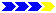Next page